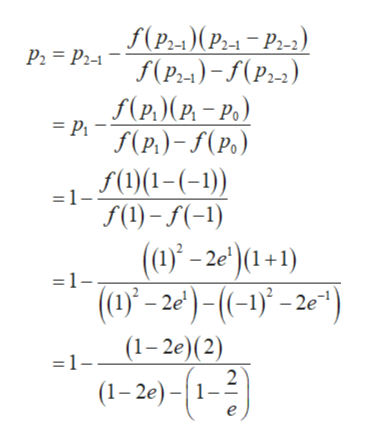# r2 -2e = 0 has a solution in the interval -1,1]6. (10 points) The equation f(x)(a) (5 points) With po = -1 and pi = 1 calculate p2 using the Secant method.(b) (5 points) With p2 from part (a) calculate p3 using Newton's method

Question
6 viewshelp_outlineImage Transcriptioncloser2 -2e = 0 has a solution in the interval -1,1] 6. (10 points) The equation f(x) (a) (5 points) With po = -1 and pi = 1 calculate p2 using the Secant method. (b) (5 points) With p2 from part (a) calculate p3 using Newton's method fullscreen
check_circle

Step 1

(a) The given equation is f(x) = x2 – 2ex = 0 has a solution in the interval [−1, 1].

The secant iteration is defined as,

Step 2

Substitute p0 = − 1 and p1 = 1 to find the value of p2 as follows.help_outlineImage TranscriptioncloseS(Р-)(Ра — Р-з) Р, — Ръя f(р) -S(P-2) S(p.)(P - Р.) = Pf(P)-(Po) Г01-(-1) 1 f(1)() (0' - 2е) (1+1) (0)-2е)-(-)ў - 2е") =1 (1-2е)(2) =1- (1-2)-1-2 fullscreen
Step 3

On further simpli...

### Want to see the full answer?

See Solution

#### Want to see this answer and more?

Solutions are written by subject experts who are available 24/7. Questions are typically answered within 1 hour.*

See Solution
*Response times may vary by subject and question.
Tagged in

### Math